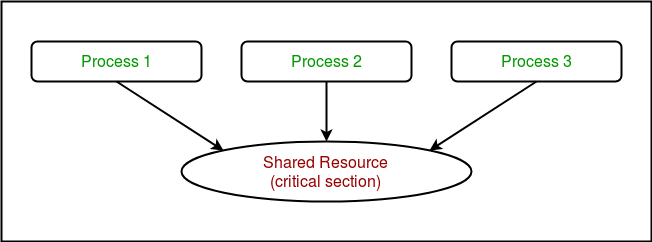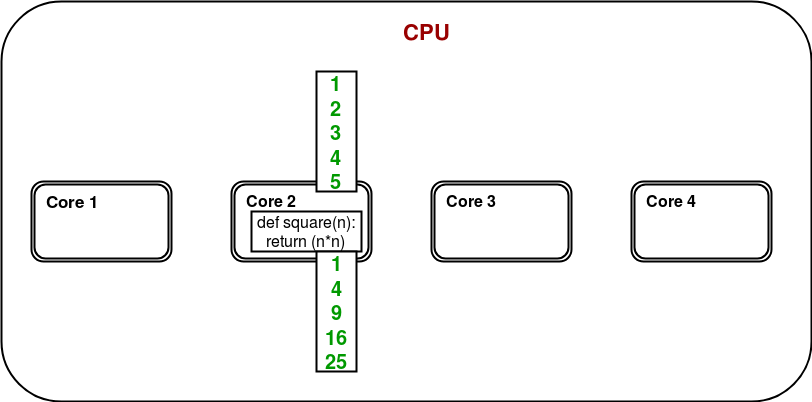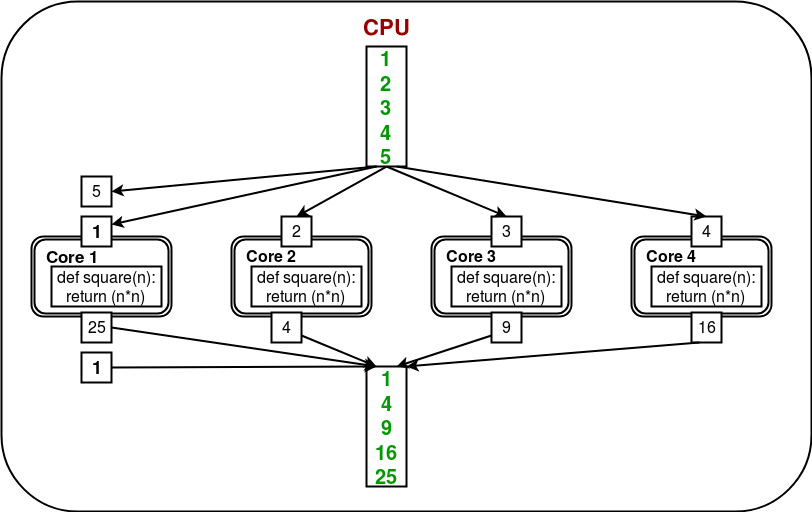# Synchronization and Pooling of processes in Python

• Difficulty Level : Medium
• Last Updated : 13 Feb, 2018

Prerequisite – Multiprocessing in Python | Set 1 , Set 2
This article discusses two important concepts related to multiprocessing in Python:

• Synchronization between processes
• Pooling of processes

Synchronization between processes

Process synchronization is defined as a mechanism which ensures that two or more concurrent processes do not simultaneously execute some particular program segment known as critical section.

Critical section refers to the parts of the program where the shared resource is accessed.

For example, in the diagram below, 3 processes try to access shared resource or critical section at the same time.Concurrent accesses to shared resource can lead to race condition.

A race condition occurs when two or more processes can access shared data and they try to change it at the same time. As a result, the values of variables may be unpredictable and vary depending on the timings of context switches of the processes.

Consider the program below to understand the concept of race condition:

 `# Python program to illustrate  ` `# the concept of race condition ` `# in multiprocessing ` `import` `multiprocessing ` ` `  `# function to withdraw from account ` `def` `withdraw(balance):     ` `    ``for` `_ ``in` `range``(``10000``): ` `        ``balance.value ``=` `balance.value ``-` `1` ` `  `# function to deposit to account ` `def` `deposit(balance):     ` `    ``for` `_ ``in` `range``(``10000``): ` `        ``balance.value ``=` `balance.value ``+` `1` ` `  `def` `perform_transactions(): ` ` `  `    ``# initial balance (in shared memory) ` `    ``balance ``=` `multiprocessing.Value(``'i'``, ``100``) ` ` `  `    ``# creating new processes ` `    ``p1 ``=` `multiprocessing.Process(target``=``withdraw, args``=``(balance,)) ` `    ``p2 ``=` `multiprocessing.Process(target``=``deposit, args``=``(balance,)) ` ` `  `    ``# starting processes ` `    ``p1.start() ` `    ``p2.start() ` ` `  `    ``# wait until processes are finished ` `    ``p1.join() ` `    ``p2.join() ` ` `  `    ``# print final balance ` `    ``print``(``"Final balance = {}"``.``format``(balance.value)) ` ` `  `if` `__name__ ``=``=` `"__main__"``: ` `    ``for` `_ ``in` `range``(``10``): ` ` `  `        ``# perform same transaction process 10 times ` `        ``perform_transactions() `

If you run above program, you will get some unexpected values like this:

```Final balance = 1311
Final balance = 199
Final balance = 558
Final balance = -2265
Final balance = 1371
Final balance = 1158
Final balance = -577
Final balance = -1300
Final balance = -341
Final balance = 157
```

In above program, 10000 withdraw and 10000 deposit transactions are carried out with initial balance as 100. The expected final balance is 100 but what we get in 10 iterations of perform_transactions function is some different values.

This happens due to concurrent access of processes to the shared data balance. This unpredictability in balance value is nothing but race condition.

Let us try to understand it better using the sequence diagrams given below. These are the different sequences which can be produced in above example for a single withdraw and deposit action.

• This is a possible sequence which gives wrong answer as both processes read the same value and write it back accordingly.
p1 p2 balance
current=100
100
current=100
100
balance=current-1=99
write(balance)
99
balance=current+1=101
write(balance)
101
• These are 2 possible sequences which are desired in above scenario.
p1 p2 balance
current=100
100
balance=current-1=99
write(balance)
99
current=99
99
balance=current+1=100
write(balance)
100
p1 p2 balance
current=100
100
balance=current+1=101
write(balance)
101
current=101
101
balance=current-1=100
write(balance)
100

Using Locks

multiprocessing module provides a Lock class to deal with the race conditions. Lock is implemented using a Semaphore object provided by the Operating System.

A semaphore is a synchronization object that controls access by multiple processes to a common resource in a parallel programming environment. It is simply a value in a designated place in operating system (or kernel) storage that each process can check and then change. Depending on the value that is found, the process can use the resource or will find that it is already in use and must wait for some period before trying again. Semaphores can be binary (0 or 1) or can have additional values. Typically, a process using semaphores checks the value and then, if it using the resource, changes the value to reflect this so that subsequent semaphore users will know to wait.

Consider the example given below:

 `# Python program to illustrate  ` `# the concept of locks ` `# in multiprocessing ` `import` `multiprocessing ` ` `  `# function to withdraw from account ` `def` `withdraw(balance, lock):     ` `    ``for` `_ ``in` `range``(``10000``): ` `        ``lock.acquire() ` `        ``balance.value ``=` `balance.value ``-` `1` `        ``lock.release() ` ` `  `# function to deposit to account ` `def` `deposit(balance, lock):     ` `    ``for` `_ ``in` `range``(``10000``): ` `        ``lock.acquire() ` `        ``balance.value ``=` `balance.value ``+` `1` `        ``lock.release() ` ` `  `def` `perform_transactions(): ` ` `  `    ``# initial balance (in shared memory) ` `    ``balance ``=` `multiprocessing.Value(``'i'``, ``100``) ` ` `  `    ``# creating a lock object ` `    ``lock ``=` `multiprocessing.Lock() ` ` `  `    ``# creating new processes ` `    ``p1 ``=` `multiprocessing.Process(target``=``withdraw, args``=``(balance,lock)) ` `    ``p2 ``=` `multiprocessing.Process(target``=``deposit, args``=``(balance,lock)) ` ` `  `    ``# starting processes ` `    ``p1.start() ` `    ``p2.start() ` ` `  `    ``# wait until processes are finished ` `    ``p1.join() ` `    ``p2.join() ` ` `  `    ``# print final balance ` `    ``print``(``"Final balance = {}"``.``format``(balance.value)) ` ` `  `if` `__name__ ``=``=` `"__main__"``: ` `    ``for` `_ ``in` `range``(``10``): ` ` `  `        ``# perform same transaction process 10 times ` `        ``perform_transactions() `

Output:

```Final balance = 100
Final balance = 100
Final balance = 100
Final balance = 100
Final balance = 100
Final balance = 100
Final balance = 100
Final balance = 100
Final balance = 100
Final balance = 100
```

Let us try to understand the above code step by step:

• Firstly, a Lock object is created using:
```lock = multiprocessing.Lock()
```
• Then, lock is passed as target function argument:
``` p1 = multiprocessing.Process(target=withdraw, args=(balance,lock))
p2 = multiprocessing.Process(target=deposit, args=(balance,lock))
```
• In the critical section of target function, we apply lock using lock.acquire() method. As soon as a lock is acquired, no other process can access its critical section until the lock is released using lock.release() method.
```lock.acquire()
balance.value = balance.value - 1
lock.release()
```

As you can see in the results, the final balance comes out to be 100 every time (which is the expected final result).

Pooling between processes

Let us consider a simple program to find squares of numbers in a given list.

 `# Python program to find  ` `# squares of numbers in a given list ` `def` `square(n): ` `    ``return` `(n``*``n) ` ` `  `if` `__name__ ``=``=` `"__main__"``: ` ` `  `    ``# input list ` `    ``mylist ``=` `[``1``,``2``,``3``,``4``,``5``] ` ` `  `    ``# empty list to store result ` `    ``result ``=` `[] ` ` `  `    ``for` `num ``in` `mylist: ` `        ``result.append(square(num)) ` ` `  `    ``print``(result) `

Output:

```[1, 4, 9, 16, 25]
```

It is a simple program to calculate squares of elements of a given list. In a multi-core/multi-processor system, consider the diagram below to understand how above program will work:Only one of the cores is used for program execution and it’s quite possible that other cores remain idle.

In order to utilize all the cores, multiprocessing module provides a Pool class. The Pool class represents a pool of worker processes. It has methods which allows tasks to be offloaded to the worker processes in a few different ways. Consider the diagram below:Here, the task is offloaded/distributed among the cores/processes automatically by Pool object. User doesn’t need to worry about creating processes explicitly.

Consider the program given below:

 `# Python program to understand  ` `# the concept of pool ` `import` `multiprocessing ` `import` `os ` ` `  `def` `square(n): ` `    ``print``(``"Worker process id for {0}: {1}"``.``format``(n, os.getpid())) ` `    ``return` `(n``*``n) ` ` `  `if` `__name__ ``=``=` `"__main__"``: ` `    ``# input list ` `    ``mylist ``=` `[``1``,``2``,``3``,``4``,``5``] ` ` `  `    ``# creating a pool object ` `    ``p ``=` `multiprocessing.Pool() ` ` `  `    ``# map list to target function ` `    ``result ``=` `p.``map``(square, mylist) ` ` `  `    ``print``(result) `

Output:

```Worker process id for 2: 4152
Worker process id for 1: 4151
Worker process id for 4: 4151
Worker process id for 3: 4153
Worker process id for 5: 4152
[1, 4, 9, 16, 25]
```

Let us try to understand above code step by step:

• We create a Pool object using:
``````  p = multiprocessing.Pool()
``````

There are a few arguments for gaining more control over offloading of task. These are:

• processes: specify the number of worker processes.
• maxtasksperchild: specify the maximum number of task to be assigned per child.

All the processes in a pool can be made to perform some initialization using these arguments:

• initializer: specify an initialization function for worker processes.
• initargs: arguments to be passed to initializer.
• Now, in order to perform some task, we have to map it to some function. In the example above, we map mylist to square function. As a result, the contents of mylist and definition of square will be distributed among the cores.
``````  result = p.map(square, mylist)
``````
• Once all the worker processes finish their task, a list is returned with the final result.

This article is contributed by Nikhil Kumar. If you like GeeksforGeeks and would like to contribute, you can also write an article using contribute.geeksforgeeks.org or mail your article to contribute@geeksforgeeks.org. See your article appearing on the GeeksforGeeks main page and help other Geeks.

Please write comments if you find anything incorrect, or you want to share more information about the topic discussed above.

My Personal Notes arrow_drop_up
Recommended Articles
Page :